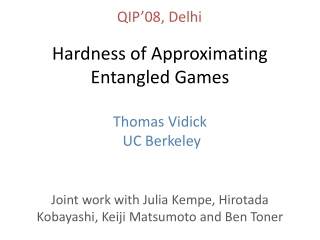DownloadDownload PresentationHardness of Approximating Entangled Games

# Hardness of Approximating Entangled Games

Download Presentation## Hardness of Approximating Entangled Games

- - - - - - - - - - - - - - - - - - - - - - - - - - - E N D - - - - - - - - - - - - - - - - - - - - - - - - - - -
##### Presentation Transcript

1. QIP’08, Delhi Hardness of Approximating Entangled Games Thomas Vidick UC Berkeley Joint work with Julia Kempe, Hirotada Kobayashi, Keiji Matsumoto and Ben Toner

2. 2-Prover Games • Verifier samples q,q’ in Q according to π • He sends q to A, q’ to B • He receives a,a’ as answers • Provers win iff V(a,a’|q,q’) = 1 Alice Bob q q’ Verifier Value of the game ω = Max. Winning Prob. (over all the prover’s strategies) Game G = (Q,A,π,V) of size |G| = poly(|Q|) a a’ accept reject

3. Alice Bob How hard is it to approximate ω? Input: game G = (Q,A,π,V) of size |G| = poly(|Q|) q’ Computing ω is NP-hard (Cook-Levin) Approximating ω is NP-hard (PCP Thm.) Approximating ω is NP-hard [Hastad’01] XOR-games • What is the situation in the quantum world? • Understand the power gained by entangled provers. How does it affect the value of a game? General classical games Alice Bob a a’ Unique games q Approximating ω is NP-hard [Khot’02] [CONJECTURE] Verifier accept reject

4. Entangled Games |ψ> The provers may share arbitrary entanglement q’ Alice Bob Value of the game ω* = Max. Winning Prob. (over all the prover’s strategies and shared |ψ>) a a’ • Provers can now produce nonlocal correlations: • - Bell inequalities 1962 • Hardness of approximating ω* is a major open question q Verifier accept reject

5. How hard is it to approximate ω*? (entangled provers) q’ Computing ω* is in P [CHTW04] (there is a semi- definite program) XOR-games What about general entangledgames? Alice Bob a a’ Unique games Can we also compute their value in P (is there an SDP?) q Approximating ω* is in P [KRT07] (there is an SDP) Verifier accept reject

6. Our Results Entangled games are hard to approximate • Theorem • There is an ε=1/poly(|Q|) such that distinguishing between ω*=1 and ω*≤1-ε is NP-hard for games with • Classical communication with three provers. • Quantum communication with two provers. • Corollary • Unless P=NP there are no SDPs of size poly(|Q|) which optimum approximates the value of entangled games (in contrast to XOR-games, unique games, single-prover quantum games). • The hierarchy of [PironioNavascuesAcin’07] cannot yield polynomial-sized SDPs (see also [DohertyLiangTonerWehner’08])

7. Outline of the proof • Do a reduction from the classical setting • Start with a classical 2-player game → NP-hard to distinguish between ω=1 and ω < 0.01 • Transform into a quantum game such that • ω=1 ω*=1 • ω<0.01 ω*<1-ε • (by construction) • (we show: ω*<1-εω<0.01) • Transform into a quantum game such that • Add tests that limit the prover’s use of entanglement • → They can do little better than using it as shared • randomness • → It is NP-hard to distinguish between ω*=1 and ω* < 1- ε

8. The modified game Alice Bob Alice Bob a a’ |a> |a’> q |q> q’ |q’> ω=1→ ω*=1 : Good provers from the classical game pass both tests. Verifier Verifier accept accept reject reject • With probability ½ do : • Classical Test : play classical game : send |q>, |q’> and check answers • Quantum Test : send |0>|q>|q’> + |1>|q’>|q> • After answer, do C-SWAP on last two registers, measure first in |±> basis, accept if |+> Quantum provers : |q> → |a>, |q’> → |a’> |0>|q>|q’> + |1>|q’>|q> → |0>|a>|a’> + |1>|a’>|a> → (|0>+|1>)|a>|a’> Classical provers : q → a, q’ → a’

9. Goal: ω*>1-εω>0.01 After you do the math… • The prover’s strategies can be described by orthogonal projectors for every q such that : • Classical test : Prob. that the verifier measures a,a’ as • answers to q,q’: • Swap-test : The W’s almost commute: → This is the key relation that we will use to round to a classical strategy

10. Special Case : ε=0 : W’s are diagonal in the same basis Set of projectors ↔ partition of space Norm of product ↔ dimension of the intersection We can easily simulate this classically by sampling using shared randomness → Classical strategy has the same success prob. as the quantum strategy

11. General case : ε = 1/poly • Are the W’s “almost diagonal” in a common basis? • If we can find Ŵ such that Ŵ≈ W and Ŵ can be simultaneously diagonalized, then we are done • “Do almost commutingmatrices nearly commute?” • Long-standing open question [Halmos’76] • Finally proven true for two hermitian matrices [Lin’97] • False for three or more hermitian matrices and the operator norm! • But… • - Our matrices have a special form (projectors) • - Our norm is not the operator norm • → It is an open question • We get around this by using a different rounding technique • → we lose a factor O(|Q|4 ε) in statistical distance

12. Hardness of computing the value of entangled games Computing ω* is in P 2-prover quantum games XOR-games 3-prover classical games Unique games Approximating ω* to within 1/poly is NP-hard Approximating ω* is in P

13. Open questions • Increase the gap! • A better gap could yield NEXP QMIP* • Prove that “almost commuting” projectors “nearly commute” • Find a better rounding procedure • Show some upper bounds • All known bounds depend on the amount of entanglement used

14. Thank you!